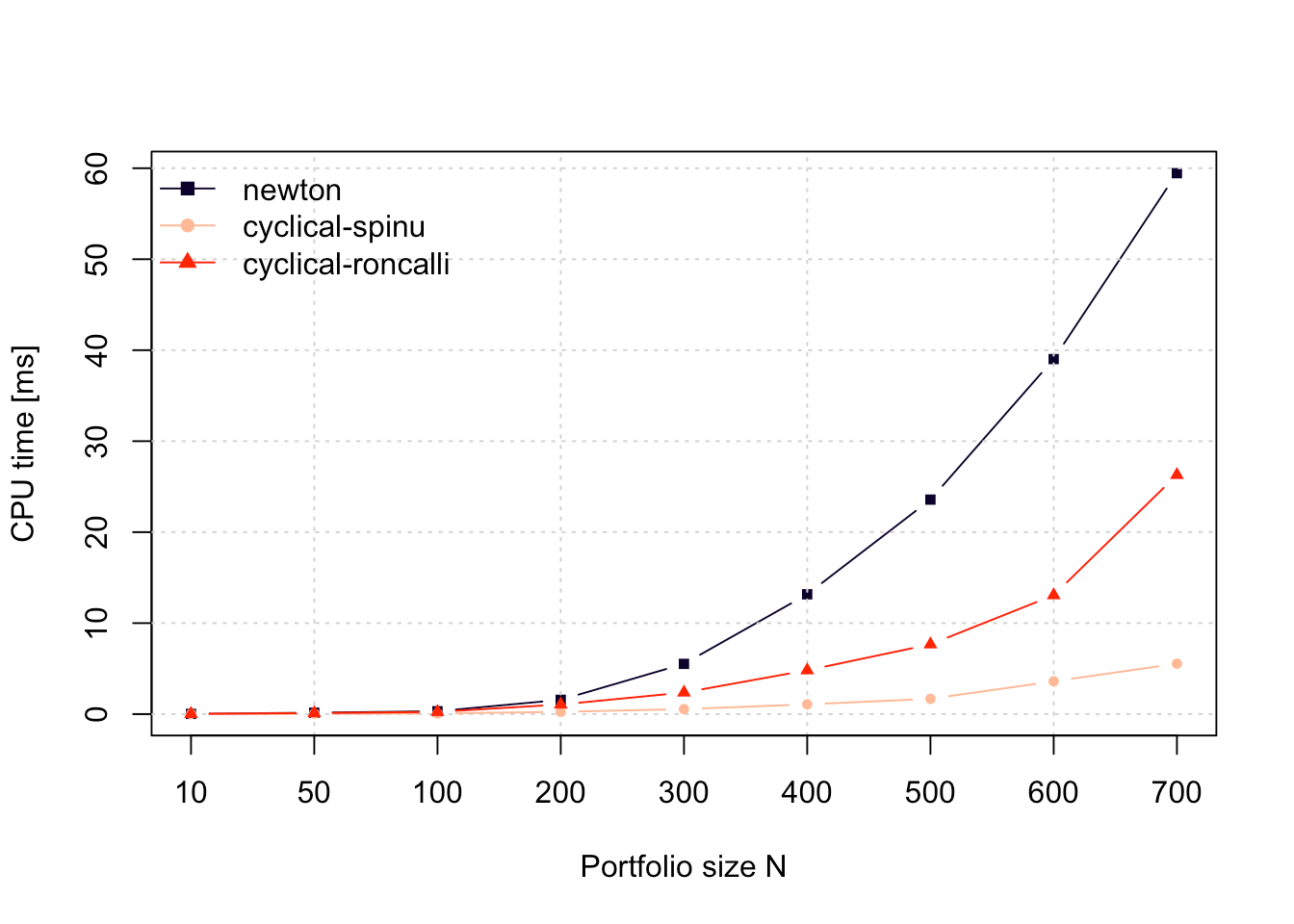This vignette illustrates the design of risk parity portfolios, widely used by practitioners in the financial industry, with the package riskParityPortfolio, gives a description of the algorithms used, and compares the performance against existing packages.

# Vanilla risk parity portfolio

A risk parity portfolio denotes a class of portfolios whose assets verify the following equalities: $w_i \dfrac{\partial f(\mathbf{w})}{\partial w_i} = w_j \dfrac{\partial f(\mathbf{w})}{\partial w_j}, \forall i, j,$ where $$f$$ is a positively homogeneous function of degree one that measures the total risk of the portfolio and $$\mathbf{w}$$ is the portfolio weight vector. In other words, the marginal risk contributions for every asset in a risk parity portfolio are equal. A common choice for $$f$$, for instance, is the standard deviation of the portfolio, which is usually called volatility, i.e., $$f(\mathbf{w}) = \sqrt{\mathbf{w}^{T}\boldsymbol{\Sigma}\mathbf{w}}$$, where $$\boldsymbol{\Sigma}$$ is the covariance matrix of the assets.

With that particular choice of $$f$$, the risk parity requirements become $w_i(\Sigma \mathbf{w})_{i} = w_j(\Sigma \mathbf{w})_{j}, \forall i, j.$

A natural extension of the risk parity portfolio is the so called risk budget portfolio, in which the marginal risk contributions match preassigned quantities. Mathematically, $w_i (\Sigma \mathbf{w})_i = b_i \mathbf{w}^{T}\Sigma\mathbf{w}, \forall i,$ where $$\mathbf{b} \triangleq (b_1, b_2, ..., b_N)$$ (with $$\mathbf{1}^T\mathbf{b}=1$$ and $$\mathbf{b}\ge\mathbf{0}$$) is the vector of desired marginal risk contributions.

In the case that $$\boldsymbol{\Sigma}$$ is diagonal and with the constraints $$\mathbf{1}^T\mathbf{w}=1$$ and $$\mathbf{w}\ge\mathbf{0}$$, the risk budgeting portfolio is $w_i = \frac{\sqrt{b_i}/\sqrt{\Sigma_{ii}}}{\sum_{k=1}^N\sqrt{b_k}/\sqrt{\Sigma_{kk}}}, \qquad i=1,\ldots,N.$

However, for non-diagonal $$\boldsymbol{\Sigma}$$ or with other additional constraints or objective function terms, a closed-form solution does not exist and some optimization procedures have to be constructed. The previous diagonal solution can always be used and is called naive risk budgeting portfolio.

With the goal of designing risk budget portfolios, Spinu proposed in  to solve the following convex optimization problem: $\underset{\mathbf{x}\ge\mathbf{0}}{\textsf{minimize}} \quad \frac{1}{2}\mathbf{x}^{T}\boldsymbol{\Sigma}\mathbf{x} - \sum_{i=1}^{N}b_i\log(x_i),$ where the portfolio can be recovered as $$\mathbf{w} = \mathbf{x}/(\mathbf{1}^T\mathbf{x})$$.

It turns out, as shown in , that the unique solution for the optimization problem stated above precisely attains the desired risk budget requirements. Such solution can be computed using convex optimization packages, such as CVXR, but faster algorithms such as Newton method and the cyclical coordinate descent method, proposed by  and , are implemented in this package.

A simple code example on how to design a risk parity portfolio is as follows:

library(riskParityPortfolio)
library(PerformanceAnalytics)  # for the color palette

# generate synthetic data
set.seed(42)
N <- 10
V <- matrix(rnorm(N^2), nrow = N)
Sigma <- cov(V)

# compute risk parity portfolio
portfolio <- riskParityPortfolio(Sigma)

# plot the portfolio designed
barplot(portfolio$w, main = "Portfolio Weights", xlab = "stocks", ylab = "dollars", beside = TRUE, col = rainbow8equal, legend = c("riskParityPortfolio"), args.legend = list(bg = "white"))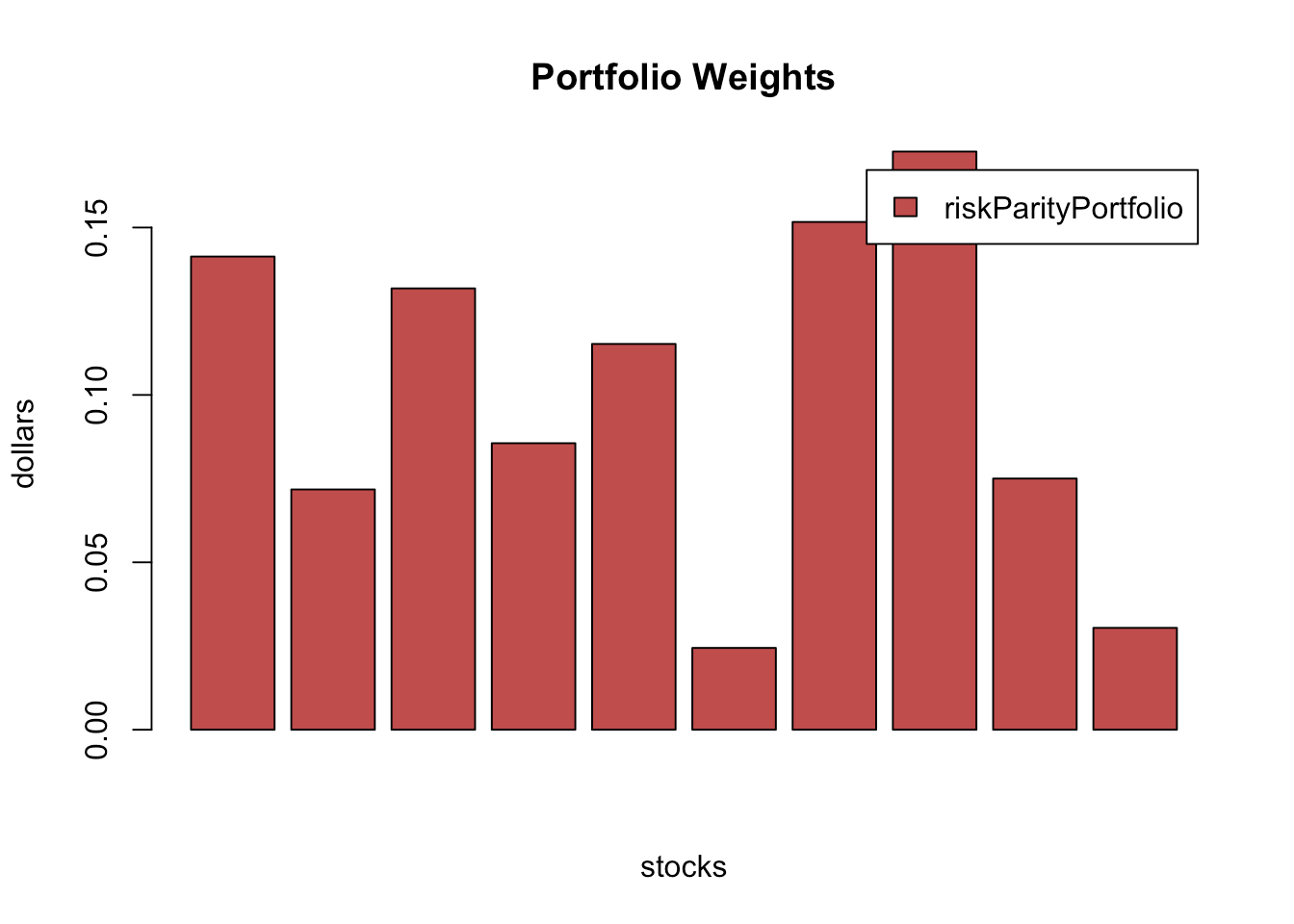# plot the risk contributions barplot(portfolio$risk_contribution,
main = "Risk Contribution of the Portfolios", xlab = "stocks", ylab = "dollars",
beside = TRUE, col = rainbow8equal, legend = c("riskParityPortfolio"),
args.legend = list(bg = "white"))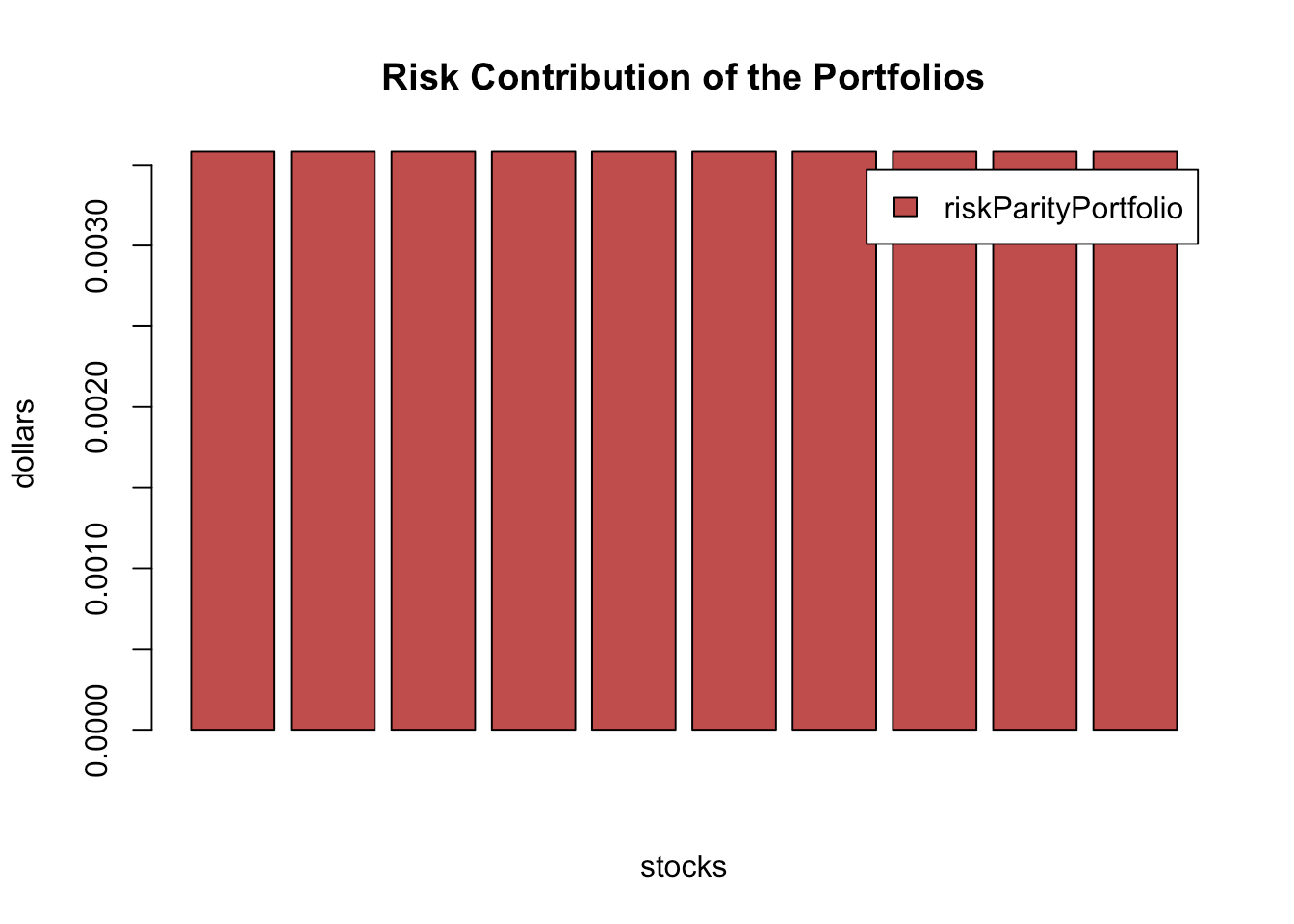As presented earlier, the risk parity portfolios are designed in such a way as to ensure equal risk contribution from the assests, which can be noted in the chart above.

# Modern risk parity portfolio

The design of risk parity portfolios as solved by  and  is of paramount importance both for academia and industry. However, practitioners would like the ability to include additional constraints and objective terms desired in practice, such as the mean return, box constraints, etc. In such cases, the risk-contribution constraints cannot be met exactly due to the trade-off among different objectives or additional constraints.

Let us explore, for instance, the effect of including the expected return as an additional objective in the optimization problem. The problem can be formulated as $\begin{array}{ll} \underset{\mathbf{w}}{\textsf{minimize}} & R(\mathbf{w}) - \lambda_{\mu} \mathbf{w}^{T}\boldsymbol{\mu}\\ \textsf{subject to} & \mathbf{1}^T\mathbf{w}=1, \mathbf{w} \ge \mathbf{0}, \end{array}$ where $$R(\mathbf{w}) = \sum_{i=1}^{N}\left(w_{i}\left(\boldsymbol{\Sigma}\mathbf{w}\right)_i - b_i\mathbf{w}^T\boldsymbol{\Sigma}\mathbf{w}\right)^{2}$$ is the risk concentration function or risk parity function, $$\mathbf{w}^{T}\boldsymbol{\mu}$$ is the expected return, and $$\lambda_{\mu}$$ is a trade-off parameter.

N <- 100
V <- matrix(rnorm(N^2), nrow = N)
Sigma <- cov(V)
mu <- runif(N)

lmd_sweep <- c(0, 10^seq(-5, 2, .25))
mean_return <- c()
risk_parity <- c()

for (lmd_mu in lmd_sweep) {
rpp <- riskParityPortfolio(Sigma, mu = mu, lmd_mu = lmd_mu,
formulation = "rc-double-index")
mean_return <- c(mean_return, rpp$mean_return) risk_parity <- c(risk_parity, rpp$risk_parity)
}

plot(risk_parity, mean_return, type = "b", pch = 19, cex = .6, col = "blue",
xlab = "Risk Parity", ylab = "Expected Return",
ylim = c(min(mean_return), max(mean_return)),
xlim = c(min(risk_parity), max(risk_parity)),
main = "Expected Return vs Risk Parity")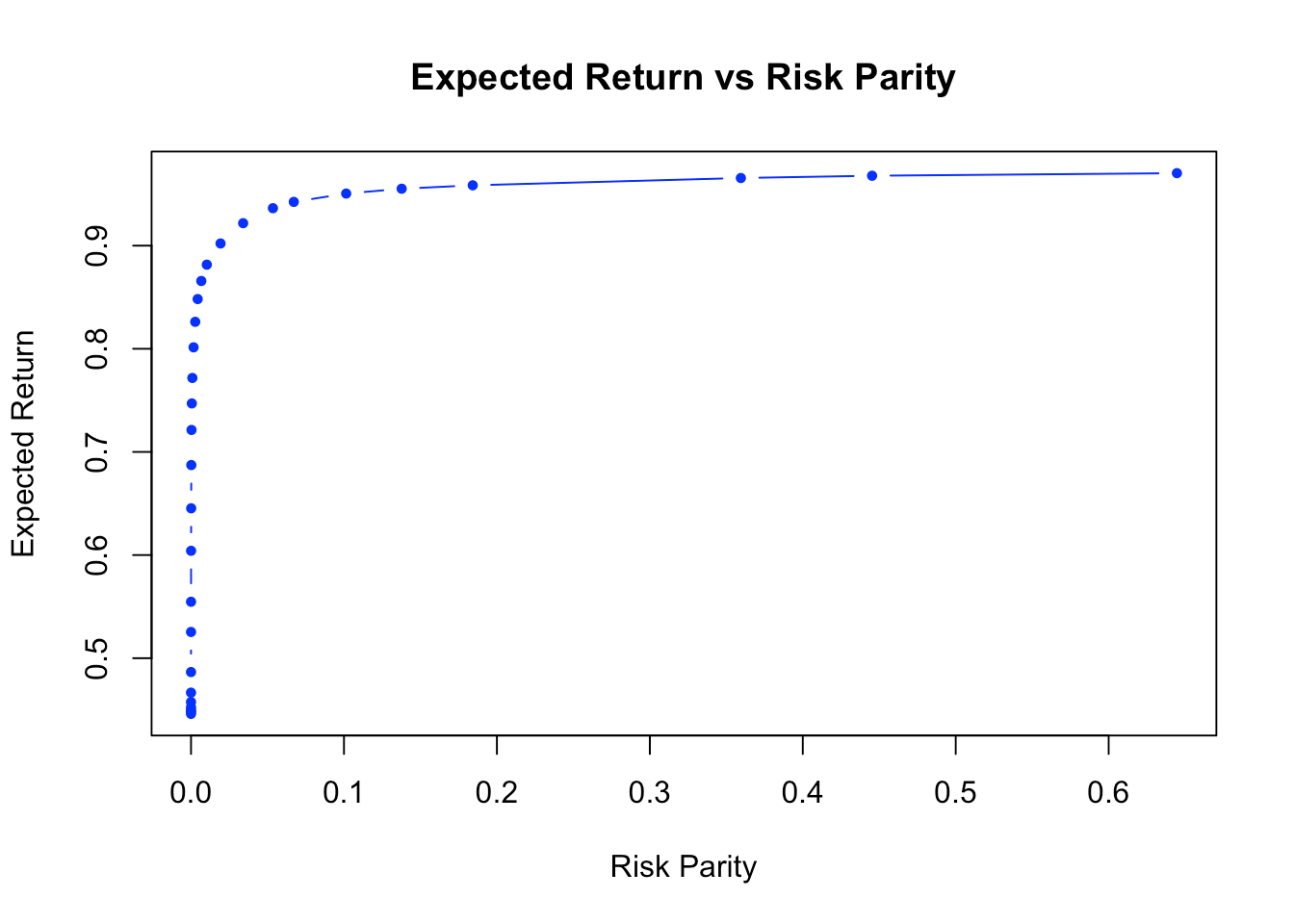Similarly, the riskParityPortfolio package allows us to include the variance as an objective term, so that the actual optimization problem can be expressed as $\begin{array}{ll} \underset{\mathbf{w}}{\textsf{minimize}} & R(\mathbf{w}) + \lambda_{\sf var} \mathbf{w}^{T}\boldsymbol{\Sigma}\mathbf{w}\\ \textsf{subject to} & \mathbf{1}^T\mathbf{w}=1, \mathbf{w} \ge \mathbf{0}, \end{array}$

In the code, that can be done by passing a positive value to the parameter lmd_var. Let’s check the following illustrative example that depicts the trade-off between volatility and risk parity:

N <- 10
Sigma <- diag(c(1:N))
lmd_sweep <- c(10 ^ (seq(-5, 5, .25)))
variance <- c()
risk_parity <- c()

for (lmd_var in lmd_sweep) {
rpp <- riskParityPortfolio(Sigma, lmd_var = lmd_var)
variance <- c(variance, rpp$variance) risk_parity <- c(risk_parity, rpp$risk_parity)
}

volatility <- sqrt(variance)
plot(risk_parity, volatility, type = "b", pch = 19, cex = .6, col = "blue",
xlab = "Risk Parity", ylab = "Volatility",
ylim = c(min(volatility), max(volatility)),
xlim = c(min(risk_parity), max(risk_parity)),
main = "Volatility vs Risk Parity")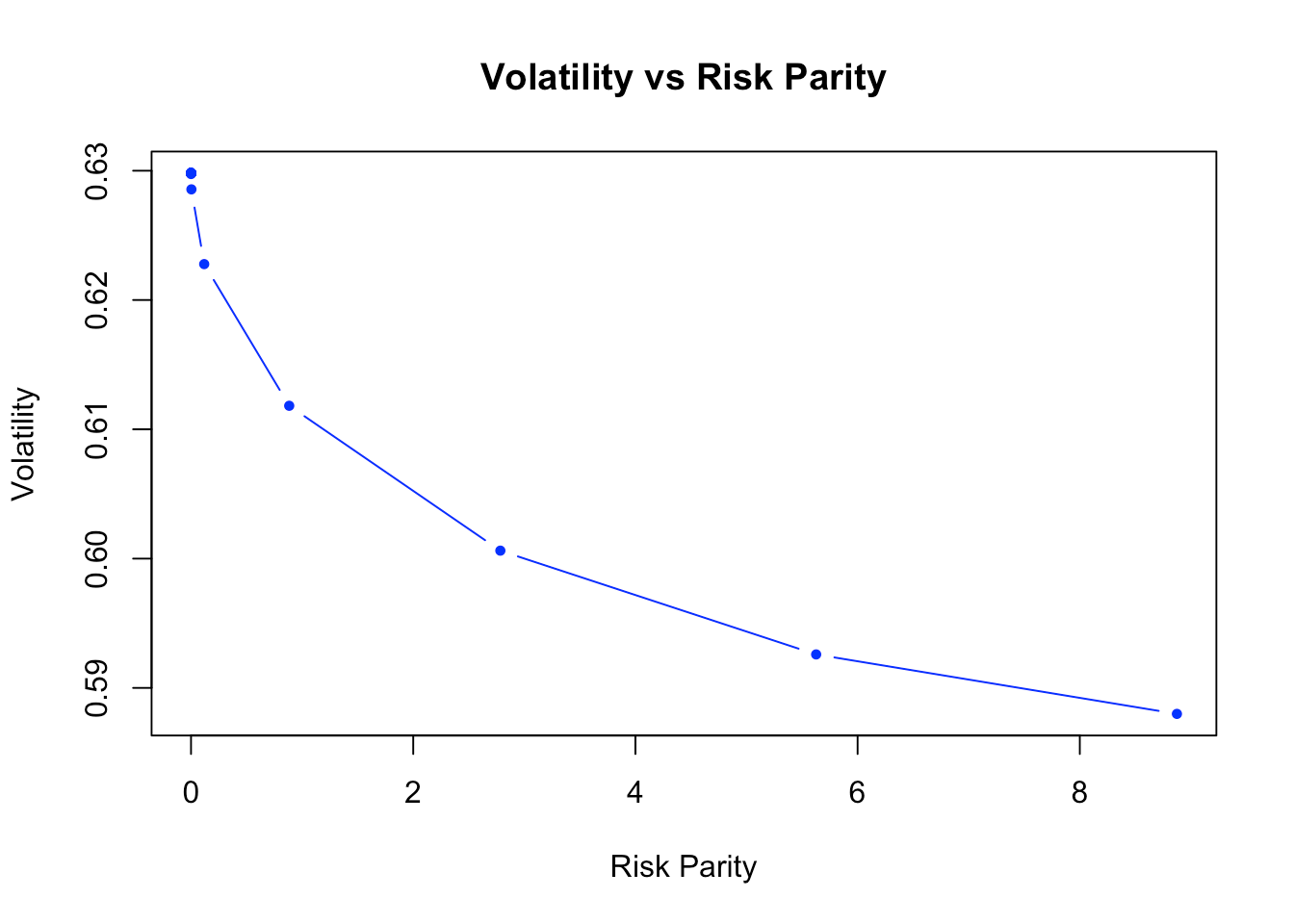# Comparison with other packages

Others R packages with the goal of designing risk parity portfolios do exist, such as FinCovRegularization, cccp, and RiskPortfolios. Let’s check how do they perform against riskParityPortfolio. (Note that other packages like FRAPO use cccp under the hood.)

library(FinCovRegularization)
library(cccp)
library(RiskPortfolios)

# generate synthetic data
set.seed(42)
N <- 10
#V <- matrix(rnorm(N^2), nrow = N)  # with this, RiskPortfolios::optimalPortfolio() fails
V <- matrix(rnorm(N*(N+N/5)), N+N/5, N)  # with this, FinCovRegularization::RiskParity() fails
Sigma <- cov(V)

# uniform initial guess for the portfolio weights
w0 <- rep(1/N, N)

# compute risk parity portfolios using different methods
rpp <- riskParityPortfolio(Sigma, w0 = w0, formulation = "rc-double-index")
#> Warning in riskParityPortfolio(Sigma, w0 = w0, formulation = "rc-double-index"): The problem is a vanilla risk parity
#> portofolio, but a nonconvex formulation has been chosen. Consider not specifying the formulation argument in order to get
#> the guaranteed global solution.
riskport_w <- optimalPortfolio(Sigma = Sigma, control = list(type = 'erc',
constraint = 'lo'))
riskport_risk_contribution <- c(riskport_w * (Sigma %*% riskport_w))
fincov_w <- RiskParity(Sigma)
fincov_risk_contribution <- c(fincov_w * (Sigma %*% fincov_w))
cccp_w <- c(getx(rp(w0, Sigma, mrc = w0, optctrl = ctrl(trace = FALSE))))
cccp_risk_contribution <- c(cccp_w * (Sigma %*% cccp_w))

barplot(rbind(rpp$w, fincov_w, cccp_w, riskport_w), main = "Portfolios Weights", xlab = "stocks", ylab = "dollars", beside = TRUE, col = rainbow8equal[1:4], legend = c("riskParityPortfolio", "FinCovRegularization", "cccp", "RiskPortfolios"), args.legend = list(bg = "white"))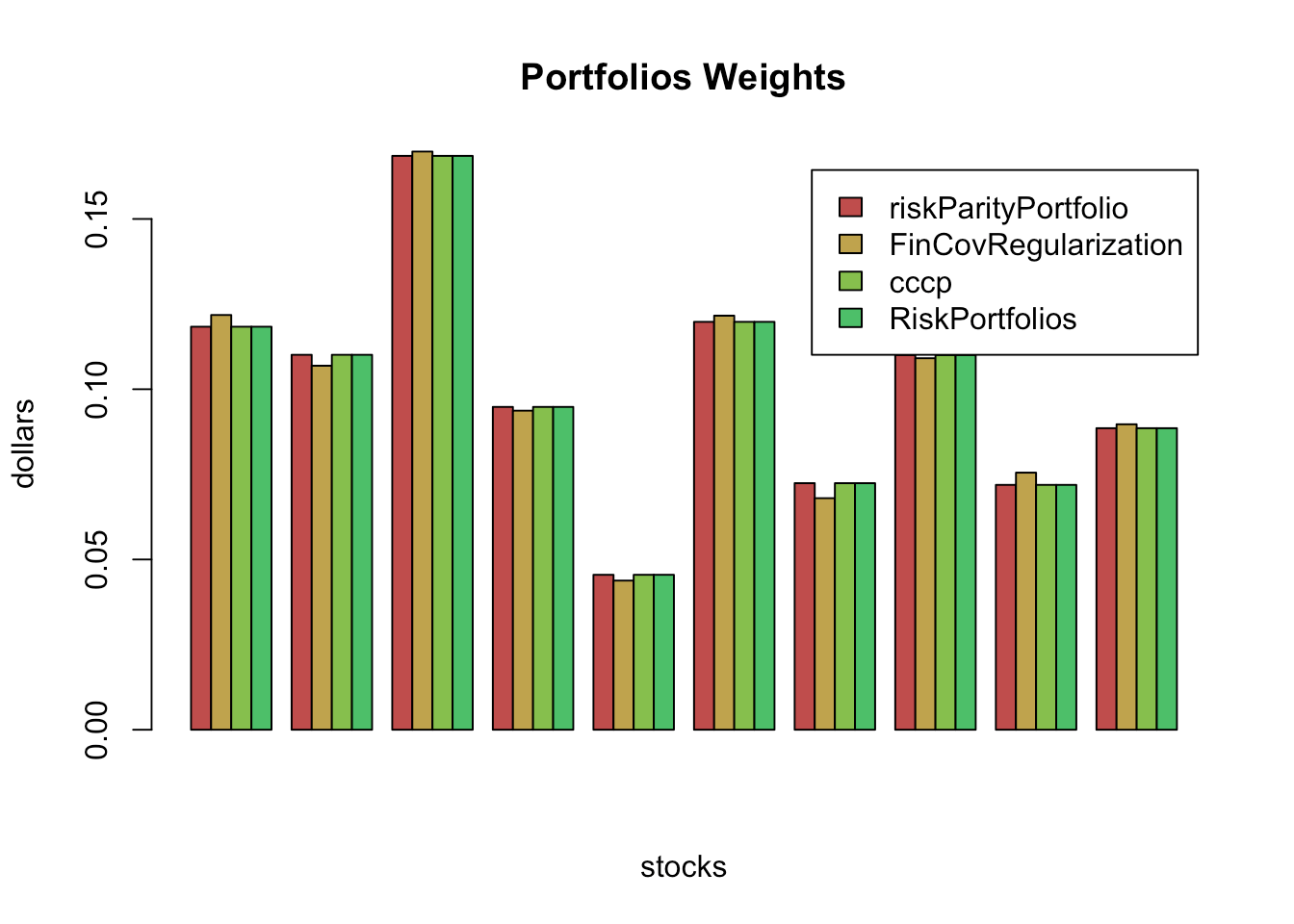barplot(rbind(rpp$risk_contribution, fincov_risk_contribution, cccp_risk_contribution,
riskport_risk_contribution),
main = "Risk Contribution of the Portfolios", xlab = "stocks", ylab = "dollars",
beside = TRUE, col = rainbow8equal[1:4],
legend = c("riskParityPortfolio", "FinCovRegularization", "cccp",
"RiskPortfolios"),
args.legend = list(x = "bottomright", bg = "white"))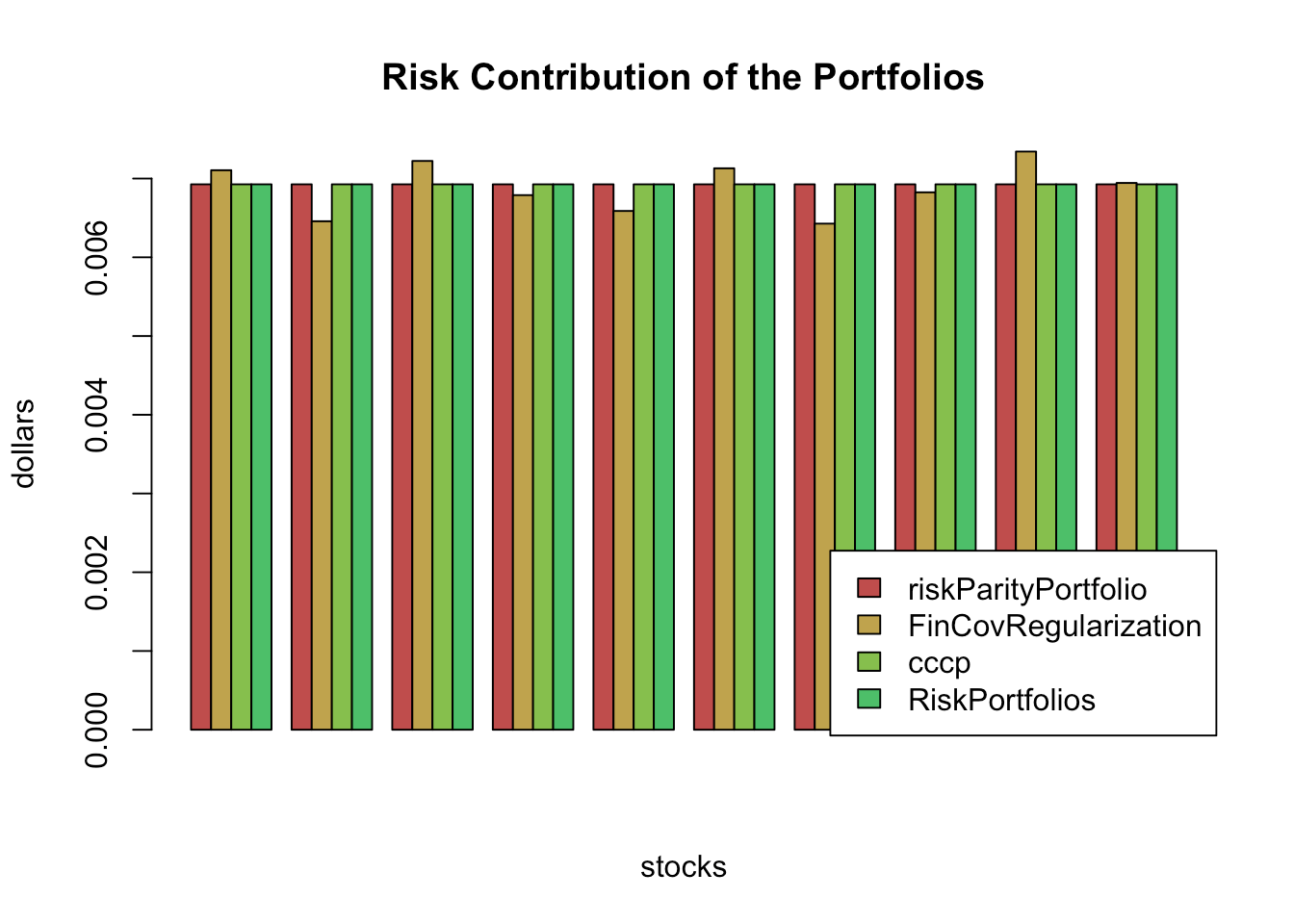Depending on the condition number of the covariance matrix, we found that the packages FinCovRegularization and RiskPortfolios may fail unexpectedly. Apart from that, the other functions perform the same.

Now, let’s see a comparison, in terms of computational time, of our cyclical coordinate descent implementation against the rp() function from the cccp package and the optimalPortfolio() function from the RiskPortfolios package. (For a fair comparison, instead of calling our function riskParityPortfolio(), we call directly the core internal function risk_parity_portfolio_ccd_spinu(), which only computes the risk parity weights, just like rp() and optimalPortfolio().)

library(microbenchmark)
library(cccp)
library(RiskPortfolios)
library(riskParityPortfolio)

N <- 100
V <- matrix(rnorm(N^2), ncol = N)
Sigma <- cov(V)
b <- rep(1/N, N)

# use risk_parity_portfolio_nn with default values of tolerance and number of iterations
op <- microbenchmark(
rp_cccp = rp(b, Sigma, b, optctrl = ctrl(trace = FALSE)),
cyclical = riskParityPortfolio:::risk_parity_portfolio_ccd_spinu(Sigma, b, 1e-6, 50),
optPort = optimalPortfolio(Sigma = Sigma, control = list(type = 'erc', constraint = 'lo')),
times = 10L)
print(op)
#> Unit: microseconds
#>      expr       min         lq       mean     median         uq        max neval
#>   rp_cccp  20848.37  21232.019  21737.382  21577.399  22188.078  23509.742    10
#>  cyclical     83.86     89.795    110.694    106.625    117.476    163.659    10
#>   optPort 780277.06 781632.180 802148.395 787964.472 813979.730 872178.975    10
par(mar = c(7, 4, 4, 2))
boxplot(op, main = "Time comparison [milliseconds]",
xlab = NULL, ylab = NULL,
unit = "ms", outline = FALSE, las = 2)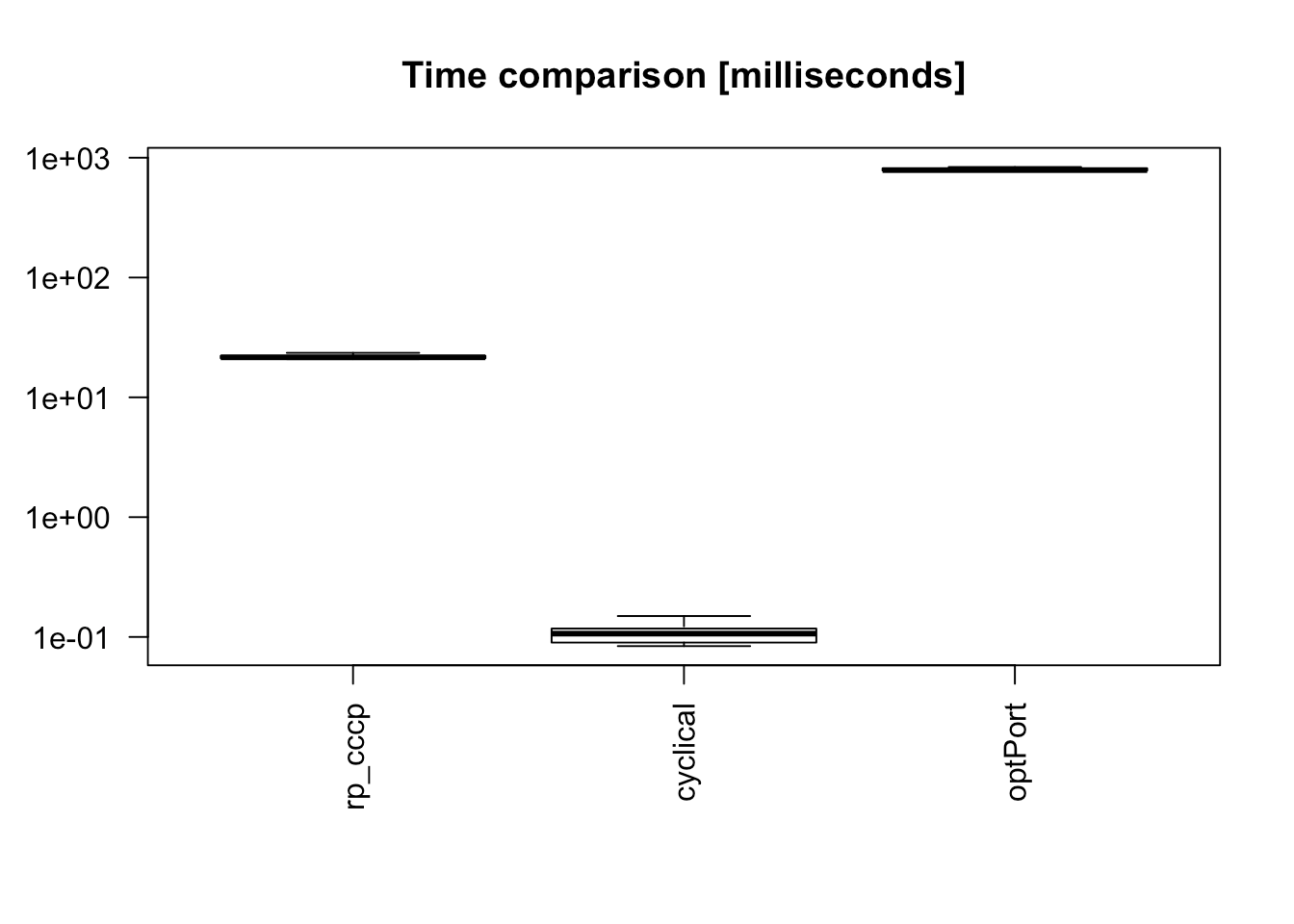As it can be observed, our implementation is orders of maginitude faster than the interior-point method used by cccp and the formulation used by RiskPortfolios.

# Appendix I: Risk concentration formulations

In general, with different constraints and objective functions, exact parity cannot be achieved and one needs to define a risk term to be minimized: $$R(\mathbf{w}) = \sum_{i=1}^{N}\left(g_{i}\left(\mathbf{w}\right)\right)^{2}$$, where the $$g_{i}$$’s denote the different risk contribution errors, e.g., $$g_{i} = w_{i}\left(\boldsymbol{\Sigma}\mathbf{w}\right)_i - b_i\mathbf{w}^T\boldsymbol{\Sigma}\mathbf{w}$$. A double-index summation can also be used: $$R(\mathbf{w}) = \sum_{i,j=1}^{N}\left(g_{ij}\left(\mathbf{w}\right)\right)^{2}$$.

We consider the risk formulations as presented in . They can be passed through the keyword argument formulation in the function riskParityPortfolio().

The name of the formulations and their mathematical expressions are presented as follows.

Formulation “rc-double-index”: $R(\mathbf{w}) = \sum_{i,j=1}^{N}\left(w_{i}\left(\boldsymbol{\Sigma}\mathbf{w}\right)_{i}-w_{j}\left(\boldsymbol{\Sigma}\mathbf{w }\right)_{j}\right)^{2}$

Formulation “rc-vs-theta”: $R(\mathbf{w},\theta) = \sum_{i=1}^{N}\left(w_{i}\left(\boldsymbol{\Sigma}\mathbf{w}\right)_i - \theta \right)^{2}$

Formulation “rc-over-var-vs-b”: $R(\mathbf{w}) = \sum_{i=1}^{N}\left(\frac{w_{i}\left(\boldsymbol{\Sigma}\mathbf{w}\right)_i}{\mathbf{w}^T\boldsymbol{\Sigma}\mathbf{w}}-b_i\right)^{2}$

Formulation “rc-over-b double-index”: $R(\mathbf{w}) = \sum_{i,j=1}^{N}\left(\frac{w_i\left(\boldsymbol{\Sigma}\mathbf{w}\right)_i}{b_i} - \frac{w_j\left(\boldsymbol{\Sigma}\mathbf{w}\right)_j}{b_j}\right)^{2}$

Formulation “rc-vs-b-times-var”: $R(\mathbf{w}) = \sum_{i=1}^{N}\left(w_{i}\left(\boldsymbol{\Sigma}\mathbf{w}\right)_i - b_i\mathbf{w}^T\boldsymbol{\Sigma}\mathbf{w}\right)^{2}$

Formulation “rc-over-sd vs b-times-sd”: $R(\mathbf{w}) = \sum_{i=1}^{N}\left(\frac{w_{i}\left(\boldsymbol{\Sigma}\mathbf{w}\right)_i}{\sqrt{\mathbf{w}^T\boldsymbol{\Sigma}\mathbf{w}}}-b_i\sqrt{\mathbf{w}^T\boldsymbol{\Sigma}\mathbf{w}}\right)^{2}$

Formulation “rc-over-b vs theta”: $R(\mathbf{w},\theta) = \sum_{i=1}^{N}\left(\frac{w_{i}\left(\boldsymbol{\Sigma}\mathbf{w}\right)_i}{b_i} - \theta \right)^{2}$

Formulation “rc-over-var”: $R(\mathbf{w}) = \sum_{i=1}^{N}\left(\frac{w_{i}\left(\boldsymbol{\Sigma}\mathbf{w}\right)_i}{\mathbf{w}^T\boldsymbol{\Sigma}\mathbf{w}}\right)^{2}$

# Appendix II: Numerical algorithms for the risk parity portfolio

In this appendix we describe the algorithms implemented for both the vanilla risk parity portfolio and the modern risk parity portfolio that may contain additional objective terms and constraints.

## Algorithms for the vanilla risk parity formulation

We now describe the implementation of the Newton method and the cyclical (coordinate) descent algorithm for the vanilla risk parity formulations presented in  and .

Consider the risk budgeting equations $w_i\left(\boldsymbol{\Sigma}\mathbf{w}\right)_i = b_i \;\mathbf{w}^{T}\boldsymbol{\Sigma}\mathbf{w}, \qquad i=1,\ldots,N$ with $$\mathbf{1}^T\mathbf{w}=1$$ and $$\mathbf{w} \ge \mathbf{0}$$.

If we define $$\mathbf{x}=\mathbf{w}/\sqrt{\mathbf{w}^{T}\boldsymbol{\Sigma}\mathbf{w}}$$, then we can rewrite the risk budgeting equations compactly as $\boldsymbol{\Sigma}\mathbf{x} = \mathbf{b}/\mathbf{x}$ with $$\mathbf{x} \ge \mathbf{0}$$ and we can always recover the portfolio by normalizing: $$\mathbf{w} = \mathbf{x}/(\mathbf{1}^T\mathbf{x})$$.

Spinu  realized that precisely that equation corresponds to the gradient of the function $$f(\mathbf{x}) = \frac{1}{2}\mathbf{x}^{T}\boldsymbol{\Sigma}\mathbf{x} - \mathbf{b}^T\log(\mathbf{x})$$ set to zero, which is the optimality condition for its minimization.

So we can finally formulate the risk budgeting problem as the following convex optimization problem: $\underset{\mathbf{x}\ge\mathbf{0}}{\textsf{minimize}} \quad \frac{1}{2}\mathbf{x}^{T}\boldsymbol{\Sigma}\mathbf{x} - \mathbf{b}^T\log(\mathbf{x}).$

Roncalli et al.  proposed a slightly different formulation (also convex): $\underset{\mathbf{x}\ge\mathbf{0}}{\textsf{minimize}} \quad \sqrt{\mathbf{x}^{T}\boldsymbol{\Sigma}\mathbf{x}} - \mathbf{b}^T\log(\mathbf{x}).$

Unfortunately, even though these two problems are convex, they do not conform with the typical classes that most solvers embrace (i.e., LP, QP, QCQP, SOCP, SDP, GP, etc.).

Nevertheless, there are several simple iterative algorithms that can be used, like the Newton method and the cyclical coordinate descent algorithm.

### Newton method

The Newton method obtains the iterates based on the gradient $$\nabla f$$ and the Hessian $${\sf H}$$ of the objective function $$f(\mathbf{x})$$ as follows: $\mathbf{x}^{(k+1)} = \mathbf{x}^{(k)} - {\sf H}^{-1}(\mathbf{x}^{(k)})\nabla f(\mathbf{x}^{(k)})$

In practice, one may need to use the backtracking method to properly adjust the step size of each iteration .

• For the function $$f(\mathbf{x}) = \frac{1}{2}\mathbf{x}^{T}\boldsymbol{\Sigma}\mathbf{x} - \mathbf{b}^T\log(\mathbf{x})$$, the gradient and Hessian are given by $\begin{array}{ll} \nabla f(\mathbf{x}) &= \boldsymbol{\Sigma}\mathbf{x} - \mathbf{b}/\mathbf{x}\\ {\sf H}(\mathbf{x}) &= \boldsymbol{\Sigma} + {\sf Diag}(\mathbf{b}/\mathbf{x}^2). \end{array}$

• For the function $$f(\mathbf{x}) = \sqrt{\mathbf{x}^{T}\boldsymbol{\Sigma}\mathbf{x}} - \mathbf{b}^T\log(\mathbf{x})$$, the gradient and Hessian are given by $\begin{array}{ll} \nabla f(\mathbf{x}) &= \boldsymbol{\Sigma}\mathbf{x}/\sqrt{\mathbf{x}^{T}\boldsymbol{\Sigma}\mathbf{x}} - \mathbf{b}/\mathbf{x}\\ {\sf H}(\mathbf{x}) &= \left(\boldsymbol{\Sigma} - \boldsymbol{\Sigma}\mathbf{x}\mathbf{x}^T\boldsymbol{\Sigma}/\mathbf{x}^{T}\boldsymbol{\Sigma}\mathbf{x}\right) / \sqrt{\mathbf{x}^{T}\boldsymbol{\Sigma}\mathbf{x}} + {\sf Diag}(\mathbf{b}/\mathbf{x}^2). \end{array}$

### Cyclical coordinate descent algorithm

This method simply minimizes in a cyclical manner with respect to each element of the variable $$\mathbf{x}$$ (denote $$\mathbf{x}_{-i}=[x_1,\ldots,x_{i-1},0,x_{i+1},\ldots,x_N]^T$$), while helding the other elements fixed.

• For the function $$f(\mathbf{x}) = \frac{1}{2}\mathbf{x}^{T}\boldsymbol{\Sigma}\mathbf{x} - \mathbf{b}^T\log(\mathbf{x})$$, the minimization w.r.t. $$x_i$$ is $\underset{x_i>0}{\textsf{minimize}} \quad \frac{1}{2}x_i^2\boldsymbol{\Sigma}_{ii} + x_i(\mathbf{x}_{-i}^T\boldsymbol{\Sigma}_{\cdot,i}) - b_i\log{x_i}$ with gradient $$\nabla_i f = x_i\boldsymbol{\Sigma}_{ii} + (\mathbf{x}_{-i}^T\boldsymbol{\Sigma}_{\cdot,i}) - b_i/x_i$$. Setting the gradient to zero gives us the second order equation $x_i^2\boldsymbol{\Sigma}_{ii} + x_i(\mathbf{x}_{-i}^T\boldsymbol{\Sigma}_{\cdot,i}) - b_i = 0$ with positive solution given by $x_i^\star = \frac{-(\mathbf{x}_{-i}^T\boldsymbol{\Sigma}_{\cdot,i})+\sqrt{(\mathbf{x}_{-i}^T\boldsymbol{\Sigma}_{\cdot,i})^2+ 4\boldsymbol{\Sigma}_{ii} b_i}}{2\boldsymbol{\Sigma}_{ii}}.$

• The derivation for the function $$f(\mathbf{x}) = \sqrt{\mathbf{x}^{T}\boldsymbol{\Sigma}\mathbf{x}} - \mathbf{b}^T\log(\mathbf{x})$$ follows similarly. The update for $$x_i$$ is given by $x_i^\star = \frac{-(\mathbf{x}_{-i}^T\boldsymbol{\Sigma}_{\cdot,i})+\sqrt{(\mathbf{x}_{-i}^T\boldsymbol{\Sigma}_{\cdot,i})^2+ 4\boldsymbol{\Sigma}_{ii} b_i \sqrt{\mathbf{x}^{T}\boldsymbol{\Sigma}\mathbf{x}}}}{2\boldsymbol{\Sigma}_{ii}}.$

## Successive convex approximation algorithm for the modern risk parity formulation

Many practical formulations deployed to design risk parity portfolios lead to nonconvex problems, specially when additional objective terms such as mean return or variance, or additional constraints, namely, shortselling, are taken into account. To circumvent the complications that arise in such formulations, Feng & Palomar  proposed a method called sucessive convex approximation (SCA). The SCA method works by convexifying the risk concentration term at some pre-defined point, casting the nonconvex problem into a much simpler strongly convex optimization problem. This procedure is then iterated until convergence is achieved. It is important to highlight that the SCA method always converges to a stationary point.

At the $$k$$-th iteration, the SCA method aims to solve \begin{align}\begin{array}{ll} \underset{\mathbf{w}}{\textsf{minimize}} & \sum_{i=1}^{n}\left(g_i(\mathbf{w}^k) + (\nabla g_i(\mathbf{w}^{k}))^{T}(\mathbf{w} - \mathbf{w}^{k})\right)^2 + \tau ||\mathbf{w} - \mathbf{w}^{k}||^{2}_{2} + \lambda F(\mathbf{w})\\ \textsf{subject to} & \mathbf{w}^{T}\mathbf{1} = 1, \mathbf{w} \in \mathcal{W}, \end{array}\end{align}

where the first order Taylor expasion of $$g_i(\mathbf{w})$$ has been used.

After some mathematical manipulations described in detail in , the optimization problem above can be rewritten as \begin{align}\begin{array}{ll} \underset{\mathbf{w}}{\textsf{minimize}} & \dfrac{1}{2}\mathbf{w}^{T}\mathbf{Q}^{k}\mathbf{w} + \mathbf{w}^{T}\mathbf{q}^{k} + \lambda F(\mathbf{w})\\ \textsf{subject to} & \mathbf{w}^{T}\mathbf{1} = 1, \mathbf{w} \in \mathcal{W}, \end{array}\end{align} where \begin{align} \mathbf{Q}^{k} & \triangleq 2(\mathbf{A}^{k})^{T}\mathbf{A}^{k} + \tau \mathbf{I},\\ \mathbf{q}^{k} & \triangleq 2(\mathbf{A}^{k})^{T}\mathbf{g}(\mathbf{w}^{k}) - \mathbf{Q}^{k}\mathbf{w}^{k}, \end{align} and \begin{align} \mathbf{A}^{k} & \triangleq \left[\nabla_{\mathbf{w}} g_{1}\left(\mathbf{w}^{k}\right), ..., \nabla_{\mathbf{w}} g_{n}\left(\mathbf{w}^{k}\right)\right]^{T} \\ \mathbf{g}\left(\mathbf{w}^{k}\right) & \triangleq \left[g_{1}\left(\mathbf{w}^{k}\right), ..., g_{n}\left(\mathbf{w}^{k}\right)\right]^{T}. \end{align}

The above problem is a quadratic program (QP) which can be efficiently solved by standard R libraries. Furthermore, it is straightforward that adding the mean return or variance terms still keeps the structure of the problem intact.

# Appendix III: Computational time

In the subsections that follows we explore the computational time required by method = "sca", method = "alabama", and method = "slsqp" for some of the formulations presented above. Additionally, we compare method = "alabama" and method = "slsqp" without using the gradient of the objective function.

## Experiment: formulation “rc-over-var vs b”

set.seed(42)
N <- 100
V <- matrix(rnorm(N^2), ncol = N)
Sigma <- V %*% t(V)
w0 <- riskParityPortfolio(Sigma, formulation = "diag")$w res_slsqp <- riskParityPortfolio(Sigma, w0 = w0, formulation = "rc-over-var vs b", method = "slsqp") #> Warning in riskParityPortfolio(Sigma, w0 = w0, formulation = "rc-over-var vs b", : The problem is a vanilla risk parity #> portofolio, but a nonconvex formulation has been chosen. Consider not specifying the formulation argument in order to get #> the guaranteed global solution. res_slsqp_nograd <- riskParityPortfolio(Sigma, w0 = w0, formulation = "rc-over-var vs b", method = "slsqp", use_gradient = FALSE) #> Warning in riskParityPortfolio(Sigma, w0 = w0, formulation = "rc-over-var vs b", : The problem is a vanilla risk parity #> portofolio, but a nonconvex formulation has been chosen. Consider not specifying the formulation argument in order to get #> the guaranteed global solution. res_alabama <- riskParityPortfolio(Sigma, w0 = w0, formulation = "rc-over-var vs b", method = "alabama") #> Warning in riskParityPortfolio(Sigma, w0 = w0, formulation = "rc-over-var vs b", : The problem is a vanilla risk parity #> portofolio, but a nonconvex formulation has been chosen. Consider not specifying the formulation argument in order to get #> the guaranteed global solution. res_alabama_nograd <- riskParityPortfolio(Sigma, w0 = w0, formulation = "rc-over-var vs b", method = "alabama", use_gradient = FALSE) #> Warning in riskParityPortfolio(Sigma, w0 = w0, formulation = "rc-over-var vs b", : The problem is a vanilla risk parity #> portofolio, but a nonconvex formulation has been chosen. Consider not specifying the formulation argument in order to get #> the guaranteed global solution. res_sca <- riskParityPortfolio(Sigma, w0 = w0, formulation = "rc-over-var vs b", method = "sca") #> Warning in riskParityPortfolio(Sigma, w0 = w0, formulation = "rc-over-var vs b", : The problem is a vanilla risk parity #> portofolio, but a nonconvex formulation has been chosen. Consider not specifying the formulation argument in order to get #> the guaranteed global solution. plot(res_slsqp_nograd$elapsed_time, res_slsqp_nograd$obj_fun, type = "b", pch=19, cex=.6, col = "blue", xlab = "Elapsed time (seconds)", ylab = "Objective function", main = "Convergence trend versus CPU time", ylim = c(0, 0.01)) lines(res_alabama$elapsed_time, res_alabama$obj_fun, type = "b", pch=18, cex=.8, col = "red") lines(res_alabama_nograd$elapsed_time, res_alabama_nograd$obj_fun, type = "b", pch=17, cex=.8, col = "purple") lines(res_slsqp$elapsed_time, res_slsqp$obj_fun, type = "b", pch=16, cex=.8, col = "green") lines(res_sca$elapsed_time, res_sca$obj_fun, type = "b", pch=15, cex=.8, col = "black") legend("topright", legend=c("alabama-nograd", "alabama", "slsqp-nograd", "slsqp", "sca"), col=c("purple", "red", "blue", "green", "black"), lty=c(1, 1, 1), cex=0.8, bg = "white")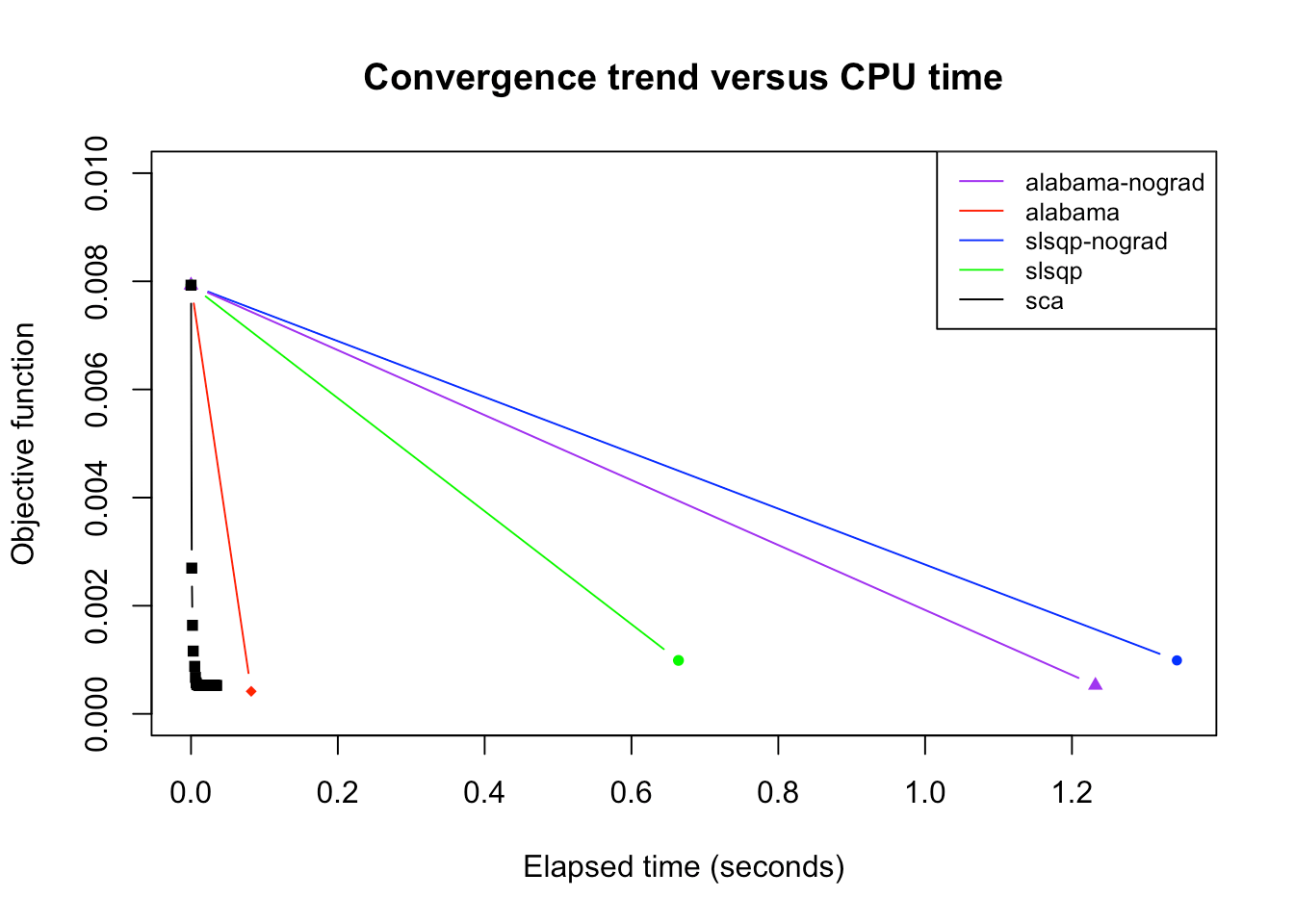## Experiment: formulation “rc vs b-times-var” res_slsqp <- riskParityPortfolio(Sigma, w0 = w0, formulation = "rc vs b-times-var", method = "slsqp") #> Warning in riskParityPortfolio(Sigma, w0 = w0, formulation = "rc vs b-times-var", : The problem is a vanilla risk parity #> portofolio, but a nonconvex formulation has been chosen. Consider not specifying the formulation argument in order to get #> the guaranteed global solution. res_slsqp_nograd <- riskParityPortfolio(Sigma, w0 = w0, formulation = "rc vs b-times-var", method = "slsqp", use_gradient = FALSE) #> Warning in riskParityPortfolio(Sigma, w0 = w0, formulation = "rc vs b-times-var", : The problem is a vanilla risk parity #> portofolio, but a nonconvex formulation has been chosen. Consider not specifying the formulation argument in order to get #> the guaranteed global solution. res_alabama <- riskParityPortfolio(Sigma, w0 = w0, formulation = "rc vs b-times-var", method = "alabama") #> Warning in riskParityPortfolio(Sigma, w0 = w0, formulation = "rc vs b-times-var", : The problem is a vanilla risk parity #> portofolio, but a nonconvex formulation has been chosen. Consider not specifying the formulation argument in order to get #> the guaranteed global solution. res_alabama_nograd <- riskParityPortfolio(Sigma, w0 = w0, formulation = "rc vs b-times-var", method = "alabama", use_gradient = FALSE) #> Warning in riskParityPortfolio(Sigma, w0 = w0, formulation = "rc vs b-times-var", : The problem is a vanilla risk parity #> portofolio, but a nonconvex formulation has been chosen. Consider not specifying the formulation argument in order to get #> the guaranteed global solution. res_sca <- riskParityPortfolio(Sigma, w0 = w0, formulation = "rc vs b-times-var", method = "sca") #> Warning in riskParityPortfolio(Sigma, w0 = w0, formulation = "rc vs b-times-var", : The problem is a vanilla risk parity #> portofolio, but a nonconvex formulation has been chosen. Consider not specifying the formulation argument in order to get #> the guaranteed global solution. plot(res_slsqp_nograd$elapsed_time, res_slsqp_nograd$obj_fun, type = "b", pch=19, cex=.6, col = "blue", xlab = "Elapsed time (seconds)", ylab = "Objective function", main = "Convergence trend versus CPU time", ylim = c(0, 0.009)) lines(res_alabama$elapsed_time, res_alabama$obj_fun, type = "b", pch=18, cex=.8, col = "red") lines(res_alabama_nograd$elapsed_time, res_alabama_nograd$obj_fun, type = "b", pch=17, cex=.8, col = "purple") lines(res_slsqp$elapsed_time, res_slsqp$obj_fun, type = "b", pch=16, cex=.8, col = "green") lines(res_sca$elapsed_time, res_sca$obj_fun, type = "b", pch=15, cex=.8, col = "black") legend("topright", legend=c("alabama-nograd", "alabama", "slsqp-nograd", "slsqp", "sca"), col=c("purple", "red", "blue", "green", "black"), lty=c(1, 1, 1), cex=0.8)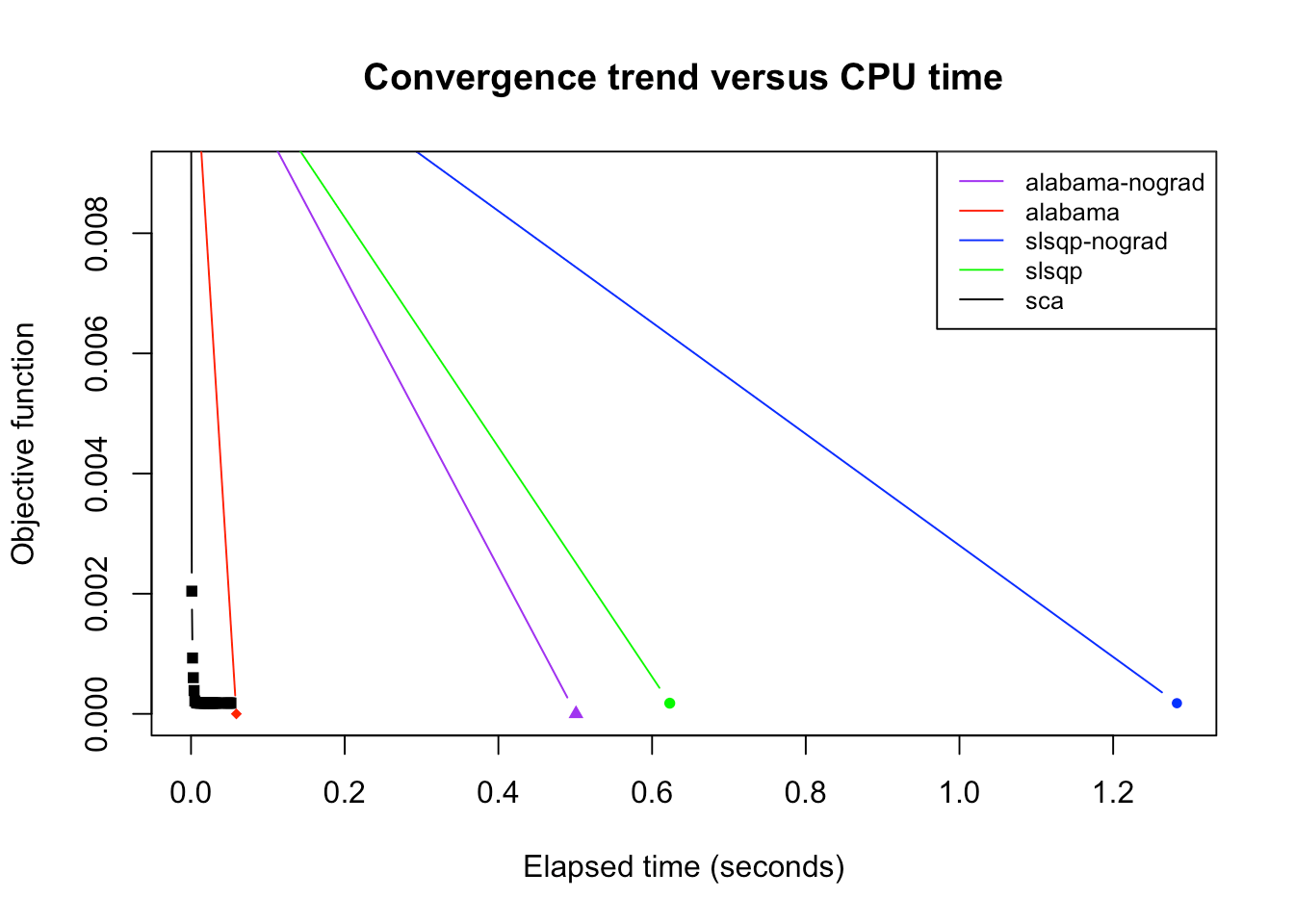## Experiment: formulation “rc-over-sd vs b-times-sd” res_slsqp <- riskParityPortfolio(Sigma, w0 = w0, formulation = "rc-over-sd vs b-times-sd", method = "slsqp") #> Warning in riskParityPortfolio(Sigma, w0 = w0, formulation = "rc-over-sd vs b-times-sd", : The problem is a vanilla risk #> parity portofolio, but a nonconvex formulation has been chosen. Consider not specifying the formulation argument in order #> to get the guaranteed global solution. res_slsqp_nograd <- riskParityPortfolio(Sigma, w0 = w0, formulation = "rc-over-sd vs b-times-sd", method = "slsqp", use_gradient = FALSE) #> Warning in riskParityPortfolio(Sigma, w0 = w0, formulation = "rc-over-sd vs b-times-sd", : The problem is a vanilla risk #> parity portofolio, but a nonconvex formulation has been chosen. Consider not specifying the formulation argument in order #> to get the guaranteed global solution. res_alabama <- riskParityPortfolio(Sigma, w0 = w0, formulation = "rc-over-sd vs b-times-sd", method = "alabama") #> Warning in riskParityPortfolio(Sigma, w0 = w0, formulation = "rc-over-sd vs b-times-sd", : The problem is a vanilla risk #> parity portofolio, but a nonconvex formulation has been chosen. Consider not specifying the formulation argument in order #> to get the guaranteed global solution. res_alabama_nograd <- riskParityPortfolio(Sigma, w0 = w0, formulation = "rc-over-sd vs b-times-sd", method = "alabama", use_gradient = FALSE) #> Warning in riskParityPortfolio(Sigma, w0 = w0, formulation = "rc-over-sd vs b-times-sd", : The problem is a vanilla risk #> parity portofolio, but a nonconvex formulation has been chosen. Consider not specifying the formulation argument in order #> to get the guaranteed global solution. res_sca <- riskParityPortfolio(Sigma, w0 = w0, formulation = "rc-over-sd vs b-times-sd", method = "sca") #> Warning in riskParityPortfolio(Sigma, w0 = w0, formulation = "rc-over-sd vs b-times-sd", : The problem is a vanilla risk #> parity portofolio, but a nonconvex formulation has been chosen. Consider not specifying the formulation argument in order #> to get the guaranteed global solution. plot(res_slsqp_nograd$elapsed_time, res_slsqp_nograd$obj_fun, type = "b", pch=19, cex=.6, col = "blue", xlab = "Elapsed time (seconds)", ylab = "Objective function", main = "Convergence trend versus CPU time", ylim = c(0, 0.01)) lines(res_alabama$elapsed_time, res_alabama$obj_fun, type = "b", pch=18, cex=.8, col = "red") lines(res_alabama_nograd$elapsed_time, res_alabama_nograd$obj_fun, type = "b", pch=17, cex=.8, col = "purple") lines(res_slsqp$elapsed_time, res_slsqp$obj_fun, type = "b", pch=16, cex=.8, col = "green") lines(res_sca$elapsed_time, res_sca$obj_fun, type = "b", pch=15, cex=.8, col = "black") legend("topright", legend=c("alabama-nograd", "alabama", "slsqp-nograd", "slsqp", "sca"), col=c("purple", "red", "blue", "green", "black"), lty=c(1, 1, 1), cex=0.8, bg = "white")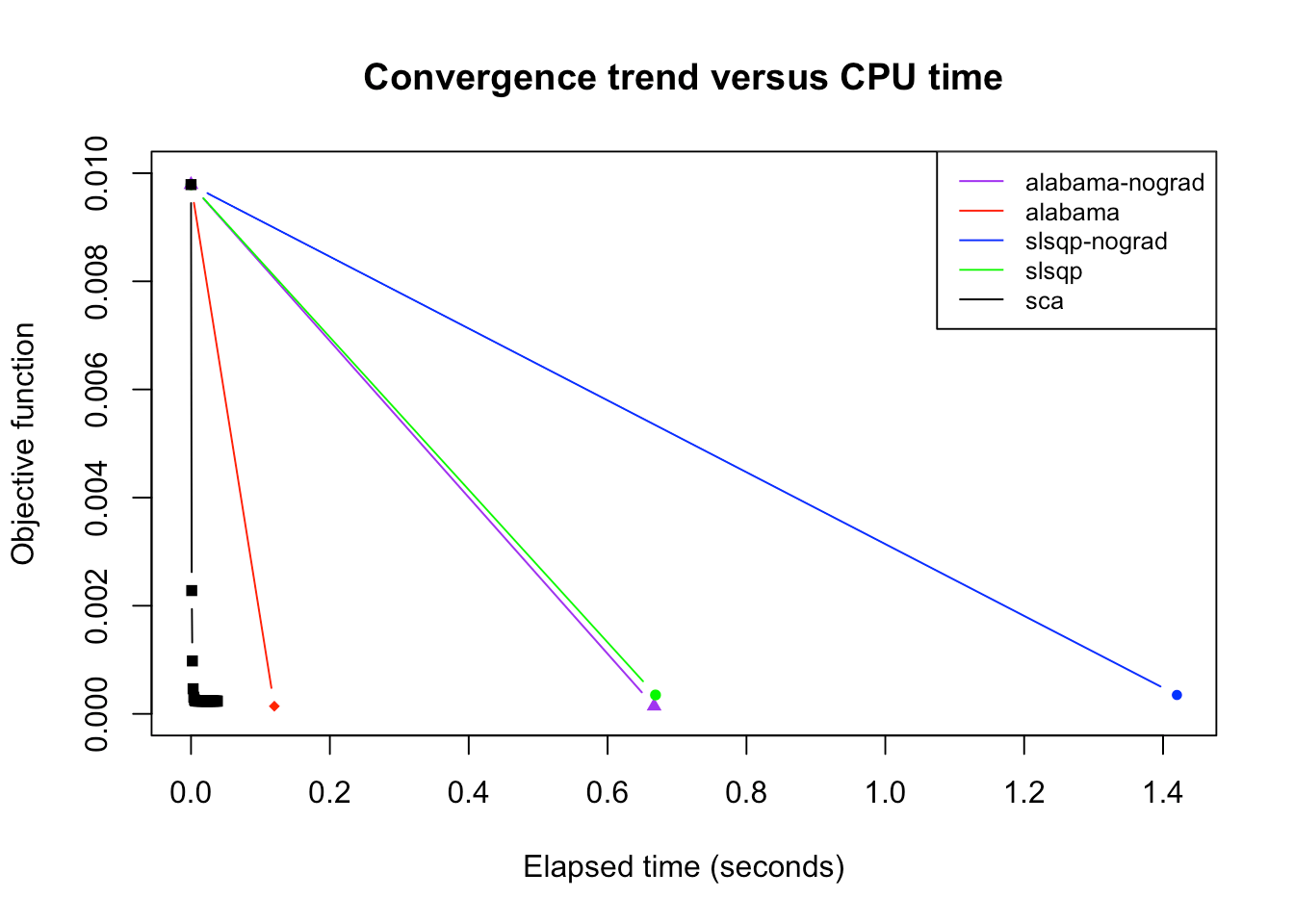## Experiment with real market data Now, let us query some real market data (from the package sparseIndexTracking) and check the time comparison of the different methods. library(sparseIndexTracking) library(xts) data(INDEX_2010) Sigma <- cov(INDEX_2010$X)
N <- nrow(Sigma)
w0 <- rep(1/N, N)

res_slsqp <- riskParityPortfolio(Sigma, w0 = w0, formulation = "rc-over-var vs b",
method = "slsqp")
#> Warning in riskParityPortfolio(Sigma, w0 = w0, formulation = "rc-over-var vs b", : The problem is a vanilla risk parity
#> portofolio, but a nonconvex formulation has been chosen. Consider not specifying the formulation argument in order to get
#> the guaranteed global solution.
res_slsqp_nograd <- riskParityPortfolio(Sigma, w0 = w0, formulation = "rc-over-var vs b",
method = "slsqp", use_gradient = FALSE)
#> Warning in riskParityPortfolio(Sigma, w0 = w0, formulation = "rc-over-var vs b", : The problem is a vanilla risk parity
#> portofolio, but a nonconvex formulation has been chosen. Consider not specifying the formulation argument in order to get
#> the guaranteed global solution.
res_alabama <- riskParityPortfolio(Sigma, w0 = w0, formulation = "rc-over-var vs b",
method = "alabama")
#> Warning in riskParityPortfolio(Sigma, w0 = w0, formulation = "rc-over-var vs b", : The problem is a vanilla risk parity
#> portofolio, but a nonconvex formulation has been chosen. Consider not specifying the formulation argument in order to get
#> the guaranteed global solution.
res_alabama_nograd <- riskParityPortfolio(Sigma, w0 = w0, formulation = "rc-over-var vs b",
method = "alabama", use_gradient = FALSE)
#> Warning in riskParityPortfolio(Sigma, w0 = w0, formulation = "rc-over-var vs b", : The problem is a vanilla risk parity
#> portofolio, but a nonconvex formulation has been chosen. Consider not specifying the formulation argument in order to get
#> the guaranteed global solution.
res_sca <- riskParityPortfolio(Sigma, w0 = w0, formulation = "rc-over-var vs b",
method = "sca")
#> Warning in riskParityPortfolio(Sigma, w0 = w0, formulation = "rc-over-var vs b", : The problem is a vanilla risk parity
#> portofolio, but a nonconvex formulation has been chosen. Consider not specifying the formulation argument in order to get
#> the guaranteed global solution.

plot(res_alabama_nograd$elapsed_time, res_alabama_nograd$obj_fun, type = "b",
pch=19, cex=.6, col = "purple", xlab = "Elapsed time (seconds)",
ylab = "Objective function", main = "Convergence trend versus CPU time")
lines(res_alabama$elapsed_time, res_alabama$obj_fun, type = "b", pch=18, cex=.8,
col = "red")
lines(res_slsqp_nograd$elapsed_time, res_slsqp_nograd$obj_fun, type = "b", pch=17,
cex=.8, col = "blue")
lines(res_slsqp$elapsed_time, res_slsqp$obj_fun, type = "b", pch=16, cex=.8,
col = "green")
lines(res_sca$elapsed_time, res_sca$obj_fun, type = "b", pch=15, cex=.8,
col = "black")

"alabama",
"slsqp",
"sca"),
col=c("purple", "red", "blue", "green", "black"), lty=c(1, 1, 1), cex=0.8,
bg = "white")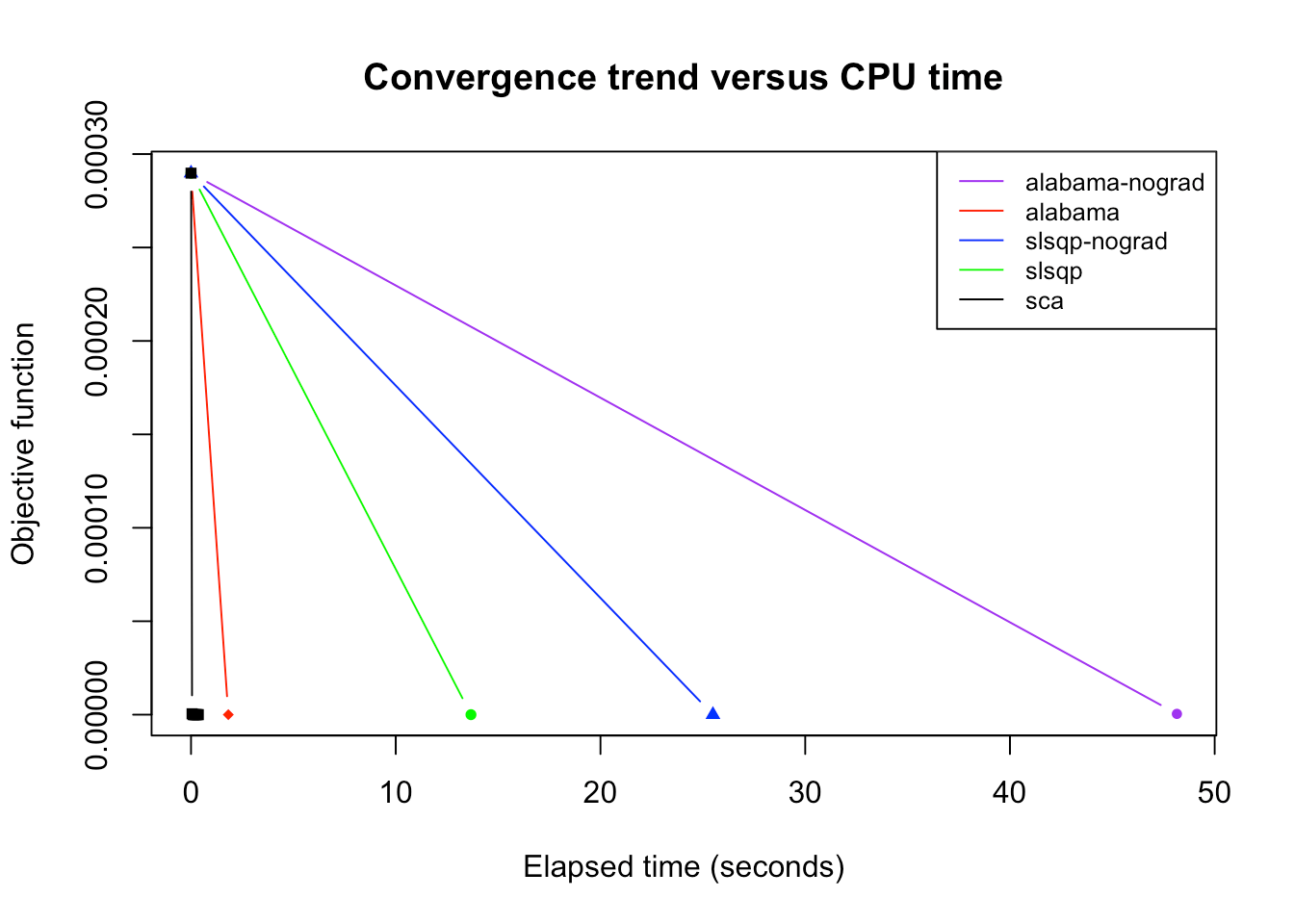It can be noted that the "alabama" and "slsqp" greatly benefit from the additional gradient information. Despite that fact, the "sca" method still performs faster. Additionally, in some cases, the "sca" method attains a better solution than the other methods.

## Design of high dimensional risk parity portfolio

In order to efficiently design high dimensional portfolios that follows the risk parity criterion, we implement the cyclical coordinate descent algorithm proposed by . In brief, this algorithm optimizes for one portfolio weight at a time while leaving the rest fixed.

The plot below illustrates the computational scaling of both Newton and cyclical algorithms. Note that the cyclical algorithm is implemented for two different formulations used by  and , respectively. Nonetheless, they output the same solution, as they should.

library(microbenchmark)
library(riskParityPortfolio)

sizes <- c(10, 50, 100, 200, 300, 400, 500, 600, 700)
size_seq <- c(1:length(sizes))
times <- matrix(0, 3, length(sizes))
for (i in size_seq) {
V <- matrix(rnorm(1000 * sizes[i]), nrow = sizes[i])
Sigma <- V %*% t(V)
bench <- microbenchmark(
newton = riskParityPortfolio(Sigma, method_init="newton"),
cyclical_spinu = riskParityPortfolio(Sigma, method_init="cyclical-spinu"),
cyclical_roncalli = riskParityPortfolio(Sigma, method_init="cyclical-roncalli"),
times = 10L, unit = "ms", control = list(order = "inorder", warmup = 4))
times[1, i] <- median(bench$time[c(TRUE, FALSE, FALSE)] / 10 ^ 6) times[2, i] <- median(bench$time[c(FALSE, TRUE, FALSE)] / 10 ^ 6)
times[3, i] <- median(bench\$time[c(FALSE, FALSE, TRUE)] / 10 ^ 6)
}

colors <- c("#0B032D", "#FFB997", "red")
plot(size_seq, times[1,], type = "b", pch=15, cex=.75, col = colors,
xlab = "Portfolio size N", ylab = "CPU time [ms]", xaxt = "n")
grid()
lines(size_seq, times[2,], type = "b", pch=16, cex=.75, col = colors)
lines(size_seq, times[3,], type = "b", pch=17, cex=.75, col = colors)
axis(side = 1, at = size_seq, labels = sizes)
legend("topleft", legend = c("newton", "cyclical-spinu", "cyclical-roncalli"),
col=colors, pch=c(15, 16, 17), lty=c(1, 1, 1), bty="n")